In this mini-lesson, we will learn about quadratic inequality, solving quadratic inequalities, quadratic inequalities in one variable,  quadratic inequalities formula,       and the graph of quadratic inequality and check out the interactive solved examples to know more about the lesson and try your hand at solving a few interesting practice questions at the end of the page.

The word “quadratic” comes from the word “quadrature”, which means "square" in Latin.

Hence, we can define a quadratic equation as second degree equation.

Therefore, a quadratic equation is also called an “Equation of degree 2”.

The general form of a quadratic equation is: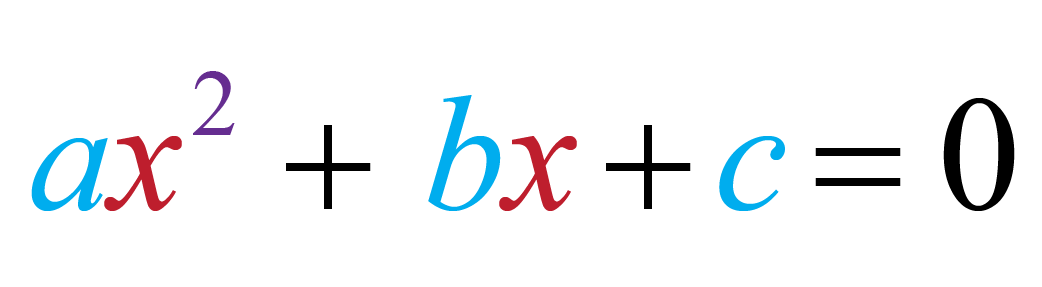In the case of a quadratic equation, the quadratic polynomial $$ax^2 + bx + c$$ is equal to zero, but what if it is not equal to zero?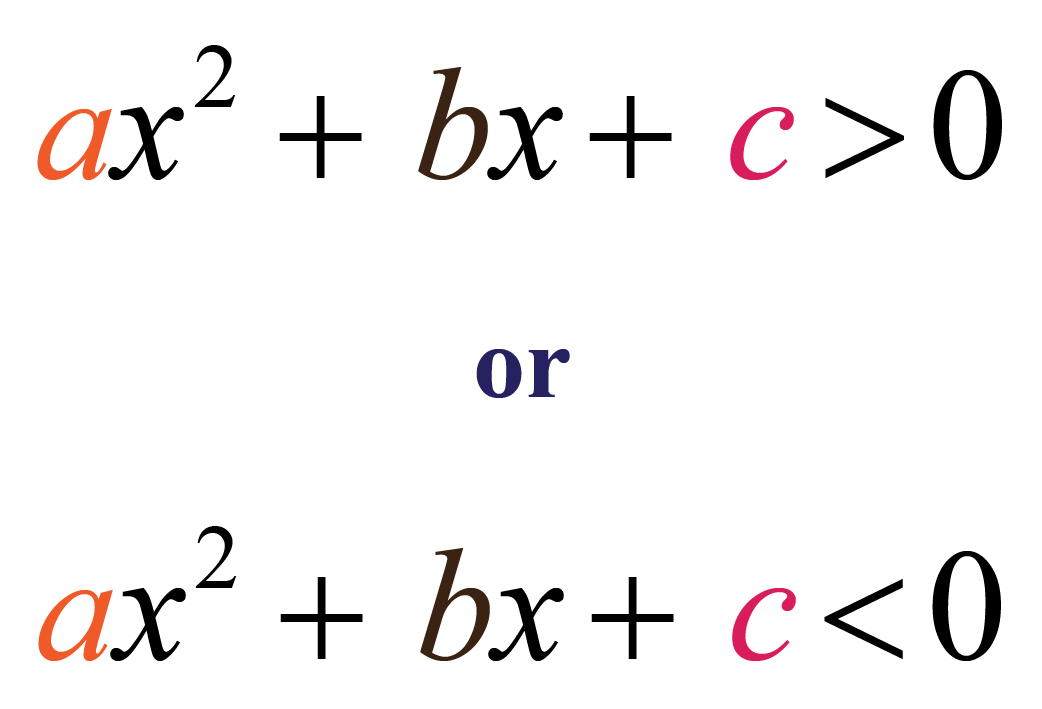The above two mathematical statements are examples of quadratic inequalities.

Now, consider the scenario where you want to build a rectangular house with a length equal to two units more than twice its breadth.If you don't want the floor area of the house to be more than 1500 $${\text{feet}}^2$$, what length and breadth can you consider?

You know that the area of a rectangle is length times its breadth.

Hence, the area of the house is $$(2 + 2x)x = 2x^2 + 2x$$, where $$x$$ is the breadth of the rectangular house.

Now, we know that the area cannot exceed 1500 $${\text{feet}}^2$$.

Thus, the quadratic inequality for the above scenario isLet's explore quadratic inequality in more detail.

## Lesson Plan

 1 What Do You Mean By Quadratic Inequalities? 2 Thinking Out of the Box! 3 Solved Examples on Quadratic Inequality 4 Challenging Questions on Quadratic Inequality 5 Interactive Questions on Quadratic inequality

## What Do You Mean By Quadratic Inequalities?

### Definition

If a quadratic polynomial in one variable is less than or greater than some number or any other polynomial (with degree less than or equal to 2), then it is said to be a quadratic inequality.

The difference between a quadratic equation and a quadratic inequality is that the quadratic equation is equal to some number while quadratic inequality is either less than or greater than some number.

Some examples of quadratic inequalities in one variable are:

$$x^2 + x - 1 > 0$$

$$2x^2 - 5x - 2 \geq 0$$

$$x^2 + 2x - 1 < 0$$

### Standard Form

The standard form of quadratic inequalities in one variable is almost the same as the standard form of a quadratic equation.

The only difference is that the quadratic equation has an "equal to" sign in it while a quadratic inequality has a "greater than" or "less than" sign (> or <).

The standard form of quadratic inequality can be represented as: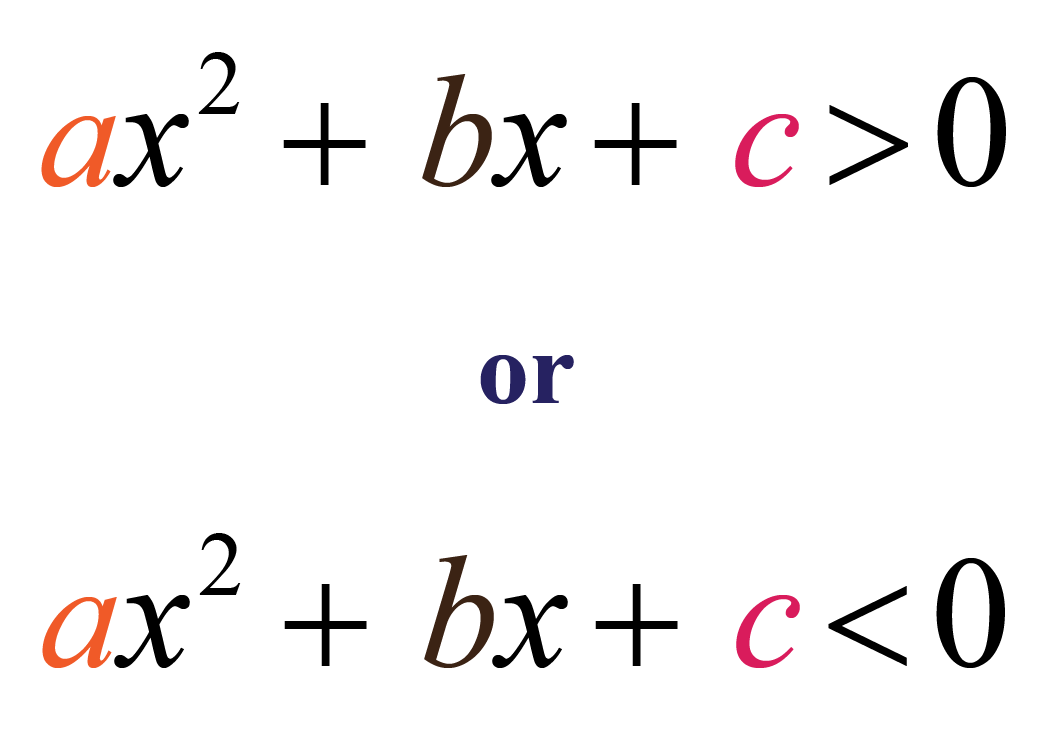## How to Solve Quadratic Inequalities?

Solving a quadratic inequality means to find the values of $$x$$ which satisfy the given condition of the question.

A quadratic second degree equation $$ax^2 + bx + c = 0$$ can have maximum 2 values of $$x$$.

But a quadratic inequality can have more than 2 values.

It can have infinite values of $$x$$ which satisfy the condition $$ax^2 + bx + c < 0$$ or $$ax^2 + bx + c > 0$$.

Solving a quadratic equation means to find the range of values of $$x$$.

Now consider a quadratic expression $$x^2 + bx + c$$.

We can write the quadratic expression in the form of $$(x - \alpha)(x - \beta)$$ and $$\alpha < \beta$$.

So by number line method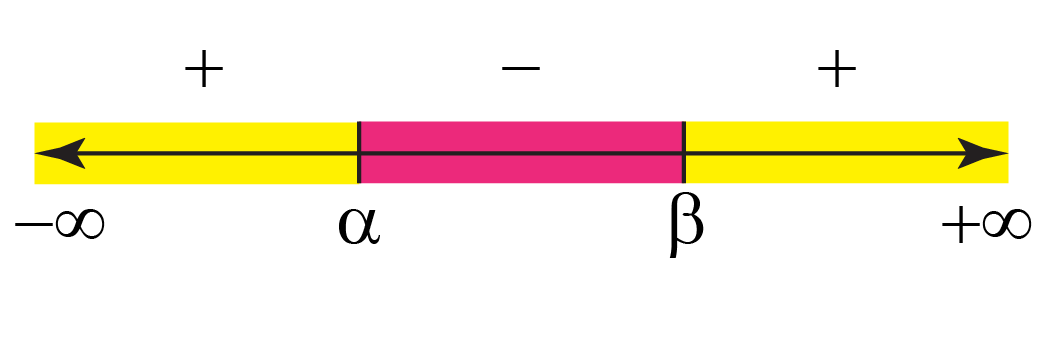It means that if $$x^2 + bx + c > 0$$, then $$x$$ can take values between $$- {\infty}$$ to $${\alpha}$$ and $${\beta}$$ to $$+ {\infty}$$.

If $$x^2 + bx + c > 0$$, then $$x \epsilon (- {\infty}, {\alpha}) \cup ({\beta}, + {\infty})$$

If $$x^2 + bx + c < 0$$, then $$x$$ can take values between $${\alpha}$$ and $${\beta}$$.

If $$x^2 + bx + c < 0$$, then $$x \epsilon ({\alpha}, {\beta})$$

Let's take a quadratic inequality $$x^2 - 1 > 0$$

$x^2 - 1 > 0 \\[0.2cm] (x - 1)(x + 1) > 0$

Here $$\alpha = -1$$ and $$\beta = 1$$

Hence, the range of $$x$$ is $$x \epsilon (- {\infty}, -1) \cup (1, + {\infty})$$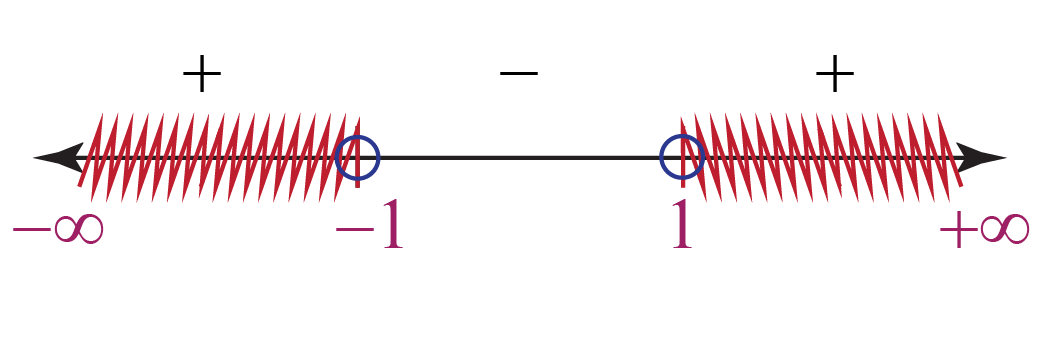If the quadratic inequality is $$x^2 - 1 < 0$$

$x^2 - 1 < 0 \\[0.2cm] (x - 1)(x + 1) < 0$

Here $$\alpha = -1$$ and $$\beta = 1$$

Hence, the range of $$x$$ is $$x \epsilon (-1, 1)$$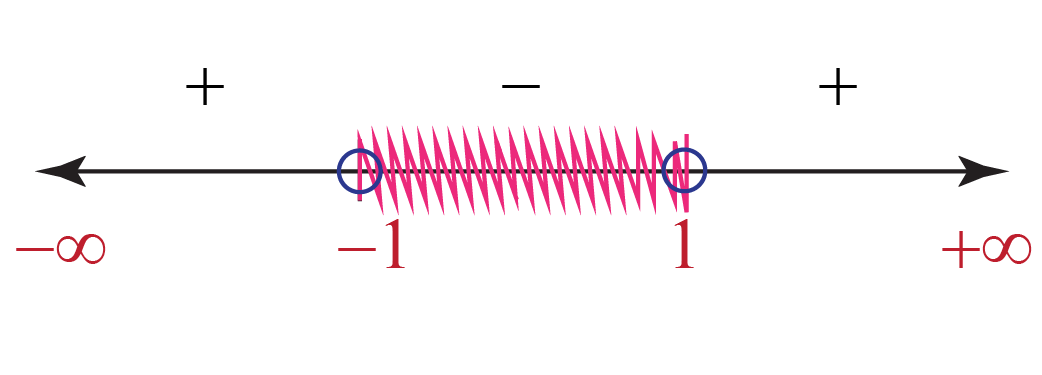If the quadratic inequality is $$x^2 - 1 \geq 0$$ (where it shows the quadratic inequality is greater than or equal to zero)

$x^2 - 1 \geq 0 \\[0.2cm] (x - 1)(x + 1) \geq 0$

Here $$\alpha = -1$$ and $$\beta = 1$$

Hence, the range of $$x$$ is $$x \epsilon (- {\infty}, -1] \cup [1, + {\infty})$$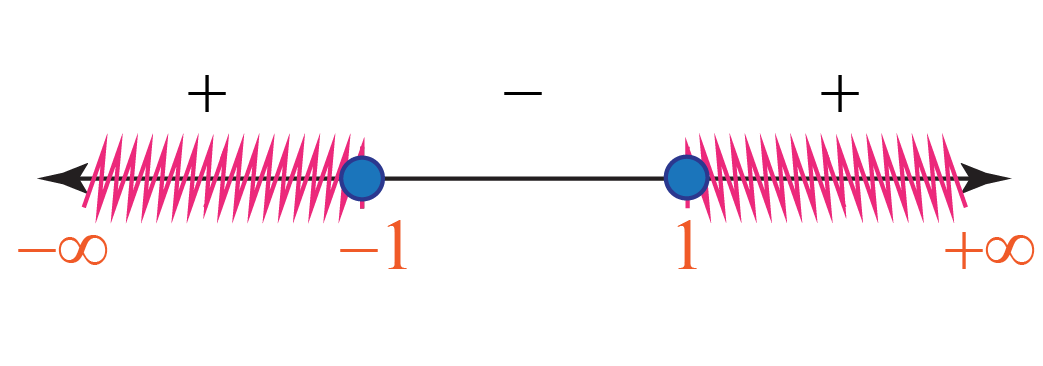If the quadratic inequality is $$x^2 - 1 \leq 0$$ (where it shows the quadratic inequality is less than or equal to zero)

$x^2 - 1 \leq 0 \\[0.2cm] (x - 1)(x + 1) \leq 0$

Here $$\alpha = -1$$ and $$\beta = 1$$

Hence, the range of $$x$$ is $$x \epsilon [-1, 1]$$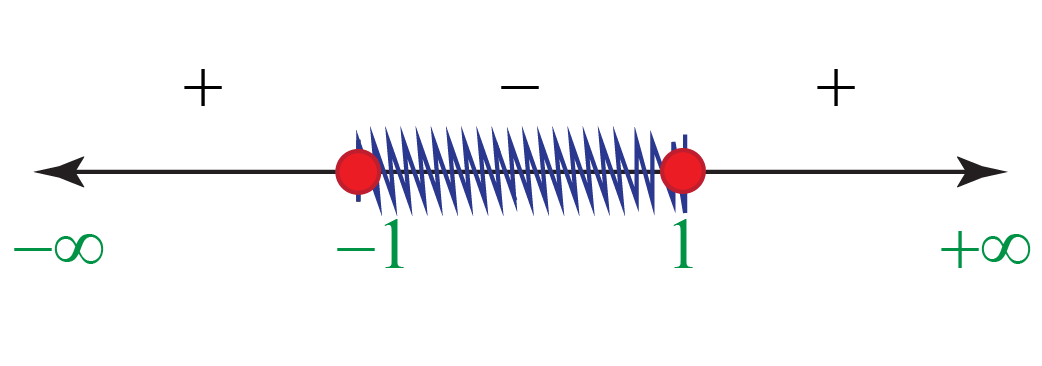Let's understand the symbols used in inequalities.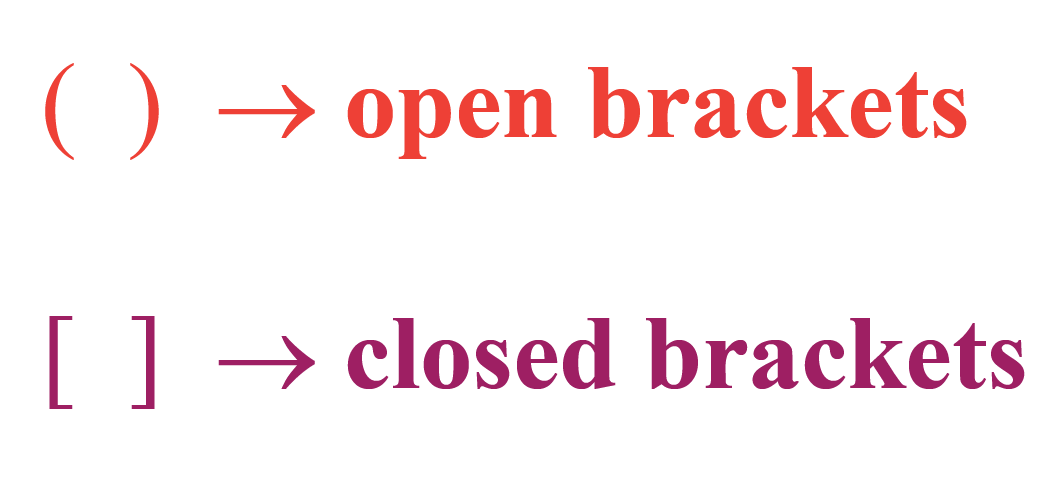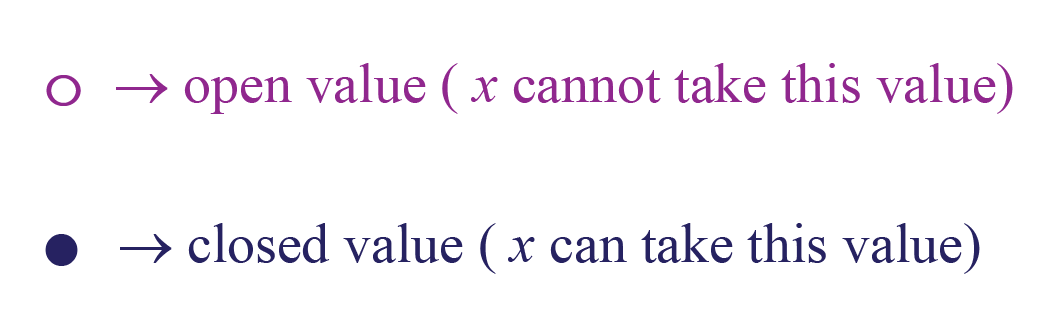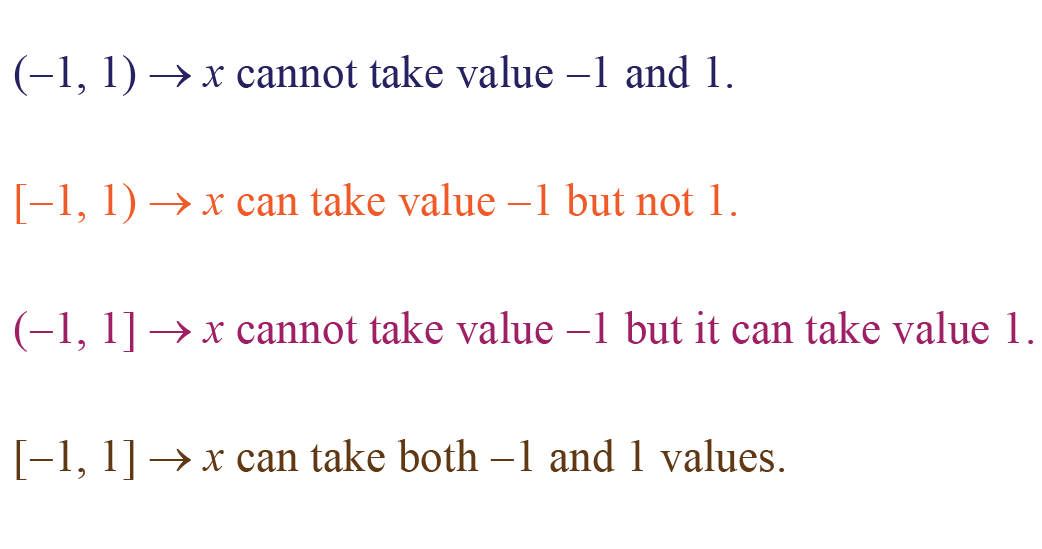Explore the quadratic inequalities calculator to find out the range of any quadratic inequality.Think Tank
• If the quadratic inequality lies in the denominator, for example, $$\dfrac{1}{(x - \alpha)(x - \beta)}$$ then how can we define the values of $$x$$ for $$\dfrac{1}{(x - \alpha)(x - \beta)} \geq 0$$? Think about it.

## Solved Examples

 Example 1

Find the range of values of $$x$$ which satisfy the inequality $$x^2 - 7x + 10 \leq 0$$.

Solution

First let's factorize the quadratic expression $$x^2 - 7x + 10$$

\begin{align} x^2 - 7x + 10 \text{ } &{\leq} \text{ } 0 \\[0.2cm] x^2 - 5x - 2x + 10 \text{ } &{\leq} \text{ } 0 \\[0.2cm] x(x - 5) - 2(x - 5) \text{ } &{\leq} \text{ } 0 \\[0.2cm] (x - 2)(x - 5) \text{ } &{\leq} \text{ } 0 \end{align}

Hence, the values of $$x$$ that satisfy the quadratic inequality are $$x \epsilon (2, 5)$$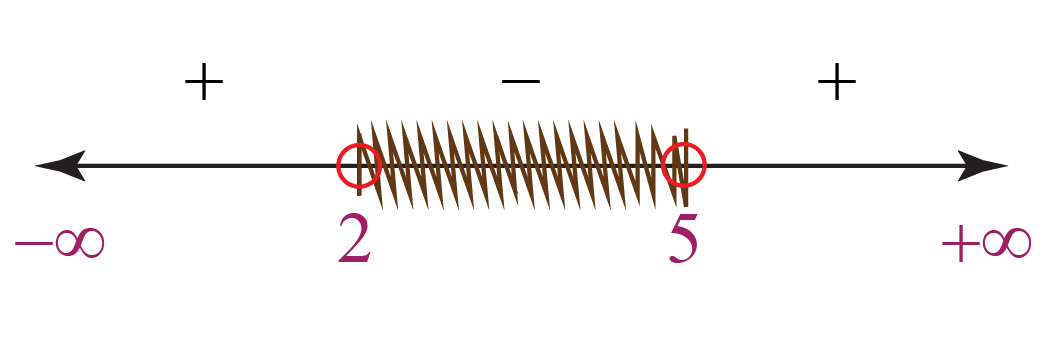$$\therefore$$ $$x \epsilon (2, 5)$$
 Example 2

Help Mathew find the range of values of $$m$$ such that the quadratic inequality $$x^2 - mx + m {\geq} 0$$ is true for all values $$x$$.

Solution

For the quadratic expression $$x^2 - mx + m$$, the coefficient of $$x^2$$ is positive, and this expression is always positive and equal to zero.

Hence, the discriminant of the quadratic equation is always negative and equal to zero.

The discriminant of the equation is $${(-m)}^2 - 4{\times}1{\times}m$$

Hence, $${(-m)}^2 - 4m {\leq} 0$$

\begin{align} {(-m)}^2 - 4m &{\leq} 0 \\[0.2cm] m^2 - 4m &{\leq} 0 \\[0.2cm] m(m - 4) &{\leq} 0 \end{align}

Thus, the values of $$m$$ that satisfy the conditions for quadratic inequality are $$m \epsilon [0, 4]$$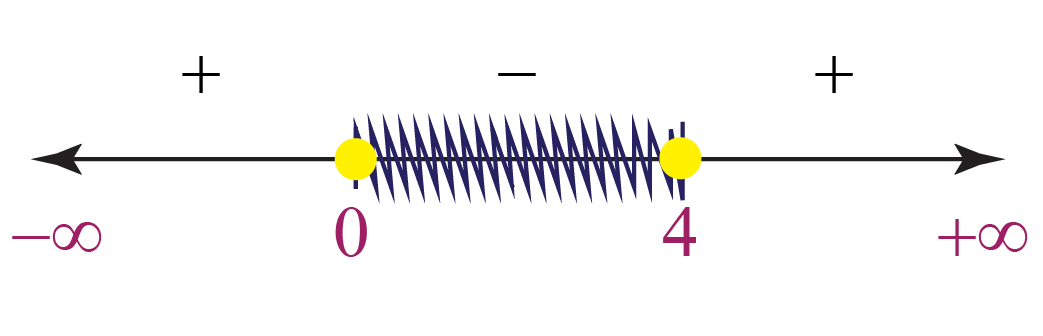$$\therefore$$ $$m \epsilon [0, 4]$$Challenging Questions
• Find the value of $$p$$ in the quadratic inequality $$2x^2 - (p + 2)x - 4 > 0$$ if $$x$$ always takes positive values.

## Interactive Questions

Here are a few activities for you to practice.

## Let's Summarize

This mini-lesson targeted the fascinating concept of quadratic inequality. The math journey around quadratic inequality starts with what a student already knows, and goes on to creatively crafting a fresh concept in the young minds. We have learned about quadratic inequality, solving quadratic inequalities, quadratic inequalities in one variable,  quadratic inequalities formula,       and the graph of quadratic inequality. Done in a way that not only it is relatable and easy to grasp, but also will stay with them forever. Here lies the magic with Cuemath.

At Cuemath, our team of math experts is dedicated to making learning fun for our favorite readers, the students!

Through an interactive and engaging learning-teaching-learning approach, the teachers explore all angles of a topic.

Be it worksheets, online classes, doubt sessions, or any other form of relation, it’s the logical thinking and smart learning approach that we, at Cuemath, believe in.

### 1. How are quadratic equations solved by the factorization method?

To solve a quadratic equation with the help of the factorization method, we split the middle term (the term with $$x$$) of the equation such that when multiplying the two numbers obtained, we get the constant term.

For example, the roots of the quadratic equation $$x^2 -3x + 2 = 0$$ by factorization method can be obtained in the following way:

\begin{align}x^2 - 3x + 2 &= 0\\[0.2cm] x^2 - 2x - x + 2 &= 0\\[0.2cm] x(x - 2) -1(x - 2) &= 0\\[0.2cm] (x - 1)(x - 2) &= 0 \end{align}

Hence, $$x = 1$$ and $$x = 2$$ are the two roots of the quadratic equation.

### 2. How do you explain solving quadratic inequalities?

Quadratic inequalities can be solved by first factorizing the quadratic expression, then finding the range of values of $$x$$ which satisfy the quadratic inequality.

You can follow this section for more details on solving quadratic inequalities.

### 3. How do you solve quadratic inequalities in two variables?

An quadratic inequalities in two variables can be solved by drawing the quadratic inequalities graph of the expression.

For example, the solutions of the inequality $$y - x^2 + 1 > 0$$ can be solved by drawing the quadratic inequalities graph of $$y = x^2 - 1$$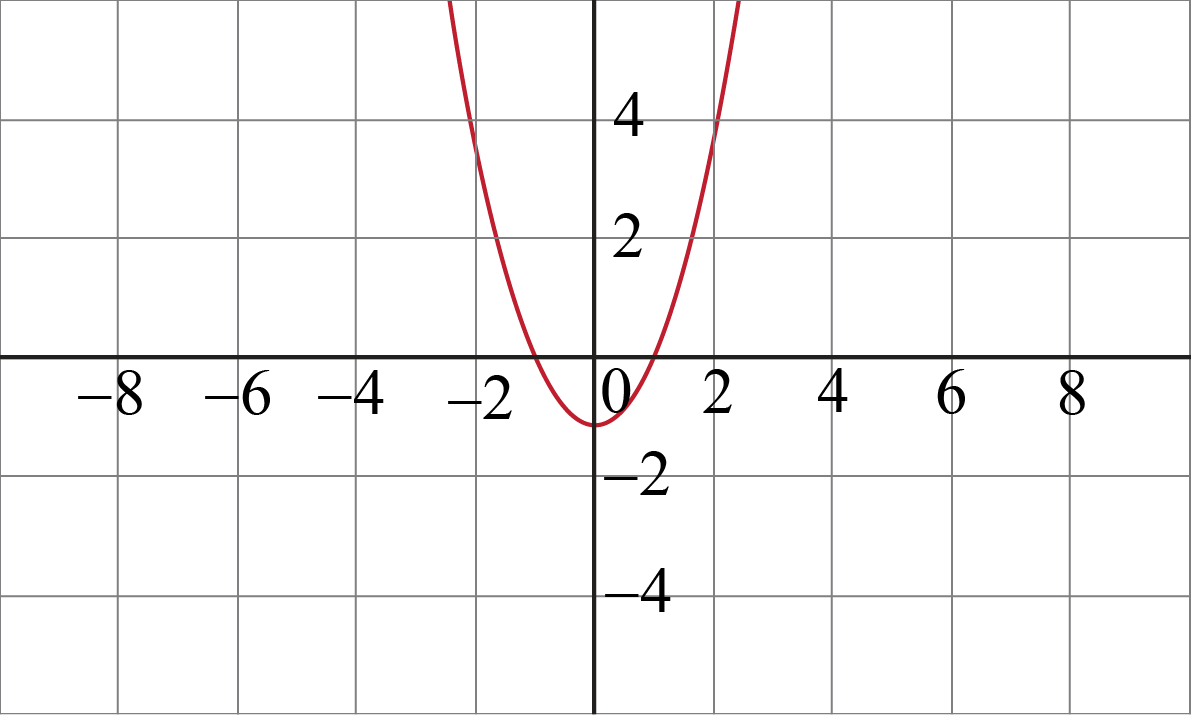From the graph, we can clearly observe that the range of $$x$$ is $$x \epsilon (-{\infty}, +{\infty})$$ and the range of $$y$$ is $$x \epsilon (-1, +{\infty})$$

### 4. How do you solve quadratic inequalities equal to zero?

If a quadratic inequality, let's say $$x^2 -5x - 6 > 0$$ is solved by equating to zero, it means that now we have to solve a quadratic equation $$x^2 -5x - 6 = 0$$.

The quadratic equation $$x^2 -5x + 6 = 0$$ can have maximum two roots.

The two values of $$x$$ which satisfy the above quadratic equations are $$x = 2$$ and $$x = 3$$.

### 5. What conditions don't affect the direction of inequality?

Adding and subtracting any number on both sides of the inequality doesn't affect the direction of the inequality.

Multiplying or dividing both sides of the inequality by a negative number changes the direction of the inequality.

Multiplying or dividing both sides of the inequality by a positive number doesn't affect the direction of the inequality.

### 6. What is the first step in solving quadratic inequality?

The first step while solving a quadratic inequality is to factorize the quadratic expression.

### 7. How do you solve quadratic inequalities word problems?

To solve a quadratic inequality word problem, first, deduce the quadratic inequality from the word problem, then solve it according to the conditions given in the question.

### 8. Can you square an inequality?

Usually, we do not prefer squaring the quadratic inequality because it might change the inequality, but for the case in which both the sides are always positive, then we can square both sides.

For example, in the quadratic inequality $$x^2 > 0$$, both sides of the inequality are always positive. Hence, we can square it.

$$x^4 > 0$$ this does not change the inequality.

### 9. How do you know if the given inequality is a quadratic inequality or not?

If the expression of the inequality is a quadratic polynomial, it will be a quadratic inequality.

### 10. What do you mean by the quadratic inequalities formula?

The quadratic inequalities formula for a quadratic equation is $$x = \dfrac{-b \pm \sqrt{b^2 - 4ac}}{2a}$$.

We can factorize the quadratic expression with the help of the above quadratic formula. Then we can solve the inequality.

grade 10 | Questions Set 2
grade 10 | Questions Set 1
More Important Topics
Numbers
Algebra
Geometry
Measurement
Money
Data
Trigonometry
Calculus
More Important Topics
Numbers
Algebra
Geometry
Measurement
Money
Data
Trigonometry
Calculus